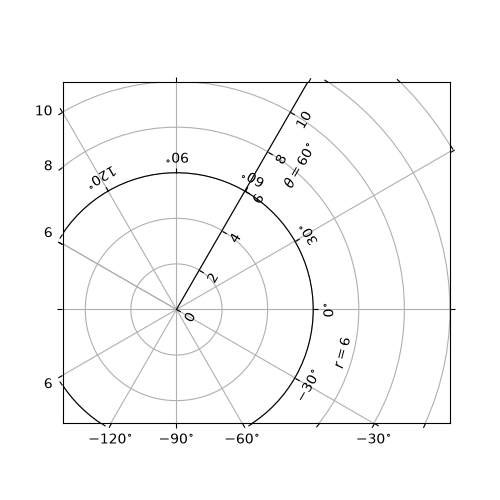# floating_axis demo#

Axis within rectangular frame.

The following code demonstrates how to put a floating polar curve within a rectangular box. In order to get a better sense of polar curves, please look at Curvilinear grid demo.import matplotlib.pyplot as plt
import numpy as np

from matplotlib.projections import PolarAxes
from matplotlib.transforms import Affine2D
from mpl_toolkits.axisartist import GridHelperCurveLinear, HostAxes
import mpl_toolkits.axisartist.angle_helper as angle_helper

def curvelinear_test2(fig):
"""Polar projection, but in a rectangular box."""
# see demo_curvelinear_grid.py for details
tr = Affine2D().scale(np.pi / 180., 1.) + PolarAxes.PolarTransform()

extreme_finder = angle_helper.ExtremeFinderCycle(20,
20,
lon_cycle=360,
lat_cycle=None,
lon_minmax=None,
lat_minmax=(0, np.inf),
)

grid_locator1 = angle_helper.LocatorDMS(12)

tick_formatter1 = angle_helper.FormatterDMS()

grid_helper = GridHelperCurveLinear(tr,
extreme_finder=extreme_finder,
grid_locator1=grid_locator1,
tick_formatter1=tick_formatter1
)

# Now creates floating axis

# floating axis whose first coordinate (theta) is fixed at 60
ax1.axis["lat"] = axis = ax1.new_floating_axis(0, 60)
axis.label.set_text(r"$\theta = 60^{\circ}$")
axis.label.set_visible(True)

# floating axis whose second coordinate (r) is fixed at 6
ax1.axis["lon"] = axis = ax1.new_floating_axis(1, 6)
axis.label.set_text(r"$r = 6$")

ax1.set_aspect(1.)
ax1.set_xlim(-5, 12)
ax1.set_ylim(-5, 10)

ax1.grid(True)

fig = plt.figure(figsize=(5, 5))
curvelinear_test2(fig)
plt.show()


Gallery generated by Sphinx-Gallery